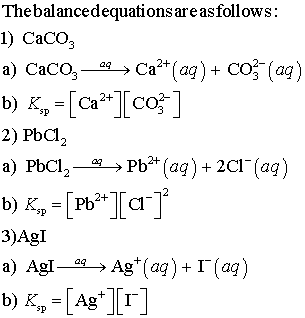# Write a balanced equation for the dissolution of CaCO3.Write an expression for Ksp for the dissolution

(1)
a. Write a balanced equation for the dissolution of CaCO3.
b. Write an expression for Ksp for the dissolution of CaCO3.

(2)
a. Write a balanced equation for the dissolution of PbCl2
b. Write an expression for Ksp for the dissolution of PbCl2.

(3)
a. Write a balanced equation for the dissolution of AgI.
b. Write an expression for Ksp for the dissolution of AgI.